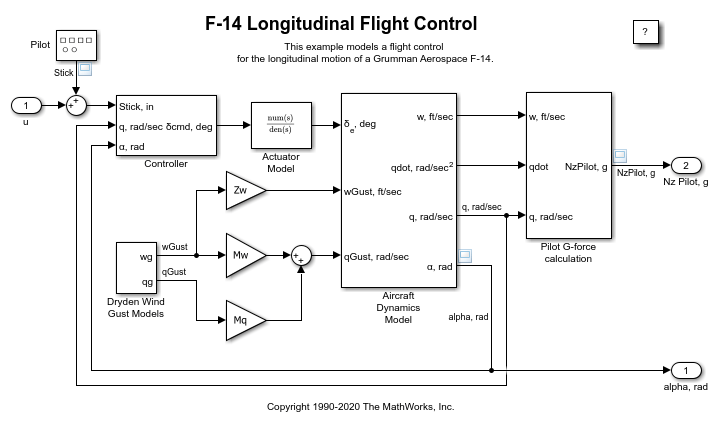Introduction to Accelerating Models

Simulink® Accelerator™ speeds up the execution of your model, by creating and compiling C code. This C code takes the place of the interpretive code that Simulink uses when in Normal mode (that is, when Simulink® is not in Accelerator mode). Simulink Accelerator generates the C code from the Simulink model and invokes the MATLAB® mex function to compile and dynamically link the generated code to Simulink. This code generation and compilation process happens the first time you accelerate the model and any time the model changes are significant enough to require re-generation (for example, the addition of a block).

This example uses the slAccelDemoF14 model to illustrate the use of Simulink Accelerator.

Measure Non-accelerated Time

Open the slAccelDemoF14 model and set the stop time to 3000 seconds. Simulate the model and time how long it takes using TIC/TOC.

modelName = 'slAccelDemoF14';
open_system(modelName)
set_param(modelName,'stoptime','3000')
tic
sim(modelName);
toc
Elapsed time is 15.441949 seconds.Turn on the Accelerator

To activate the Simulink® Accelerator, in the Simulink Editor, on the Simulation tab, under Simulate, select Accelerator. You can also issue a set_param command at the MATLAB® command prompt.

set_param(modelName,'SimulationMode','Accelerator')

Build Accelerated Model

Build the accelerated model and simulate it. When running in Accelerator mode, you can start the model by selecting Run on the Simulation tab or by running the command:

sim(modelName)

at the MATLAB prompt. In either case, the progress of the code generation process will be displayed in the MATLAB command window. To suppress this display wrap the sim command inside an evalc.

evalc('sim(modelName);');

Simulate the Accelerated Model

Simulate the model again. Notice the model simulation runs much faster on subsequent runs.

tic
sim(modelName);
toc
Elapsed time is 3.040876 seconds.

Change Tunable Parameter and Simulate Accelerated Model

Change the value of one of the tunable parameters in the model and simulate the model again. Notice the accelerated version of the model does not have to be re-generated and so the simulation still runs more quickly in Accelerated mode than in Normal mode.

modelWorkspace = get_param(modelName,'ModelWorkspace');
evalin(modelWorkspace,'Mw=Mw*2;')
tic
sim(modelName);
toc
Elapsed time is 2.597251 seconds.